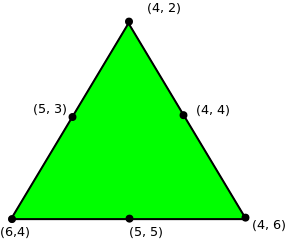# Find coordinates of the triangle given midpoint of each side

• Last Updated : 24 Nov, 2021

Given three coordinates (x, y), which are the midpoint of the sides of the triangle. The task is to find the coordinates of the triangle.

Examples:

```Input : midx1 = 5, midy1 = 3
midx2 = 4, midy2 = 4
midx3 = 5, midy3 = 5

Output : x1 = 4 y1 = 2
x2 = 4 y2 = 6
x3 = 6 y3 = 4````Solution can be verified by the figure.`

Let’s separately solve for X-coordinates and Y-coordinates. For X coordinate of vertices, let them be x1, x2, x3. Then, X-coordinate of middle points will be (x1 + x2)/2, (x2 + x3)/2, (x3 + x1)/2. Observe, sum of these 3 expressions is equal to sum of X-coordinates. Now, we have sum of 3 variables and 3 expressions for sum of every pair of them, find out the values of coordinates by solving equations.
Similarly, we solve for Y-coordinates.

Below is the implementation of this approach:

## C++

 `// C++ program to find coordinate of the``// triangle given midpoint of each side``#include``#define N 3``using` `namespace` `std;`` ` `// Return after solving the equations and``// finding the vertices coordinate.``vector<``int``> solve(``int` `v[])``{``    ``vector<``int``> res;`` ` `    ``// Finding sum of all three coordinate.``    ``int` `all3 = v + v + v;`` ` `    ``// Solving the equation.``    ``res.push_back(all3 - v*2);``    ``res.push_back(all3 - v*2);``    ``res.push_back(all3 - v*2);`` ` `    ``return` `res;``}`` ` `// Finds vertices of a triangles from given``// middle vertices.``void` `findVertex(``int` `xmid[], ``int` `ymid[])``{``    ``// Find X coordinates of vertices.``    ``vector<``int``> V1 = solve(xmid);`` ` `    ``// Find Y coordinates of vertices.``    ``vector<``int``> V2 = solve(ymid);`` ` `    ``// Output the solution.``    ``for` `(``int` `i = 0; i < 3; i++)``        ``cout << V1[i] << ``" "``             ``<< V2[i] <

## Java

 `import` `java.util.Vector;`` ` `// Java program to find coordinate of the ``// triangle given midpoint of each side ``class` `GFG {`` ` `//static final int N = 3; ``// Return after solving the equations and ``// finding the vertices coordinate. ``    ``static` `Vector solve(``int` `v[]) {``        ``Vector res = ``new` `Vector();`` ` `        ``// Finding sum of all three coordinate. ``        ``int` `all3 = v[``0``] + v[``1``] + v[``2``];`` ` `        ``// Solving the equation. ``        ``res.add(all3 - v[``1``] * ``2``);``        ``res.add(all3 - v[``2``] * ``2``);``        ``res.add(all3 - v[``0``] * ``2``);`` ` `        ``return` `res;``    ``}`` ` `// Finds vertices of a triangles from given ``// middle vertices. ``    ``static` `void` `findVertex(``int` `xmid[], ``int` `ymid[]) {``        ``// Find X coordinates of vertices. ``        ``Vector V1 = solve(xmid);`` ` `        ``// Find Y coordinates of vertices. ``        ``Vector V2 = solve(ymid);`` ` `        ``// Output the solution. ``        ``for` `(``int` `i = ``0``; i < ``3``; i++) {``            ``System.out.println(V1.get(i) + ``" "` `+ V2.get(i));``        ``}`` ` `    ``}`` ` `// Driver code ``    ``public` `static` `void` `main(String[] args) {``        ``int` `xmid[] = {``5``, ``4``, ``5``};``        ``int` `ymid[] = {``3``, ``4``, ``5``};``        ``findVertex(xmid, ymid);``    ``}``}``// This code is contributed by``// PrinciRaj1992`

## Python3

 `# Python3 program to find coordinate of the``# triangle given midpoint of each side`` ` `N ``=` `3`` ` `# Return after solving the equations and``# finding the vertices coordinate.``def` `solve(v):`` ` `    ``res ``=` `[]`` ` `    ``# Finding sum of all three coordinate.``    ``all3 ``=` `v[``0``] ``+` `v[``1``] ``+` `v[``2``]`` ` `    ``# Solving the equation.``    ``res.append(all3 ``-` `v[``1``] ``*` `2``)``    ``res.append(all3 ``-` `v[``2``] ``*` `2``)``    ``res.append(all3 ``-` `v[``0``] ``*` `2``)``    ``return` `res`` ` `# Finds vertices of a triangles from given``# middle vertices.``def` `findVertex(xmid, ymid):`` ` `    ``# Find X coordinates of vertices.``    ``V1 ``=` `solve(xmid)`` ` `    ``# Find Y coordinates of vertices.``    ``V2 ``=` `solve(ymid)`` ` `    ``# Output the solution.``    ``for` `i ``in` `range``(``0``, ``3``):``        ``print``(V1[i], end``=``" "``)``        ``print``(V2[i])`` ` `# Driver code``if` `__name__``=``=``'__main__'``:``    ``xmid ``=` `[``5``, ``4``, ``5``]``    ``ymid ``=` `[``3``, ``4``, ``5``]``    ``findVertex(xmid, ymid)`` ` `# This code is contributed by``# Sanjit_Prasad`

## C#

 `// C# program to find coordinate of the ``// triangle given midpoint of each side ``using` `System;``using` `System.Collections;`` ` `class` `GFG ``{ `` ` `    ``//static final int N = 3; ``    ``// Return after solving the equations and ``    ``// finding the vertices coordinate. ``    ``static` `ArrayList solve(``int` `[]v)``    ``{ ``        ``ArrayList res = ``new` `ArrayList(); `` ` `        ``// Finding sum of all three coordinate. ``        ``int` `all3 = v + v + v; `` ` `        ``// Solving the equation. ``        ``res.Add(all3 - v * 2); ``        ``res.Add(all3 - v * 2); ``        ``res.Add(all3 - v * 2); `` ` `        ``return` `res; ``    ``} `` ` `    ``// Finds vertices of a triangles from given ``    ``// middle vertices. ``    ``static` `void` `findVertex(``int` `[]xmid, ``int` `[]ymid)``    ``{ ``        ``// Find X coordinates of vertices. ``        ``ArrayList V1 = solve(xmid); `` ` `        ``// Find Y coordinates of vertices. ``        ``ArrayList V2 = solve(ymid); `` ` `        ``// Output the solution. ``        ``for` `(``int` `i = 0; i < 3; i++)``        ``{ ``            ``Console.WriteLine(V1[i] + ``" "` `+ V2[i]); ``        ``} `` ` `    ``} `` ` `    ``// Driver code ``    ``public` `static` `void` `Main() ``    ``{ ``        ``int` `[]xmid = {5, 4, 5}; ``        ``int` `[]ymid = {3, 4, 5}; ``        ``findVertex(xmid, ymid); ``    ``} ``} `` ` `// This code is contributed by mits`

## PHP

 ``

## Javascript

 ``

Output:

```6 4
4 2
4 6```

Time Complexity: O(1)

This article is contributed by Anuj Chauhan. If you like GeeksforGeeks and would like to contribute, you can also write an article using write.geeksforgeeks.org or mail your article to review-team@geeksforgeeks.org. See your article appearing on the GeeksforGeeks main page and help other Geeks.